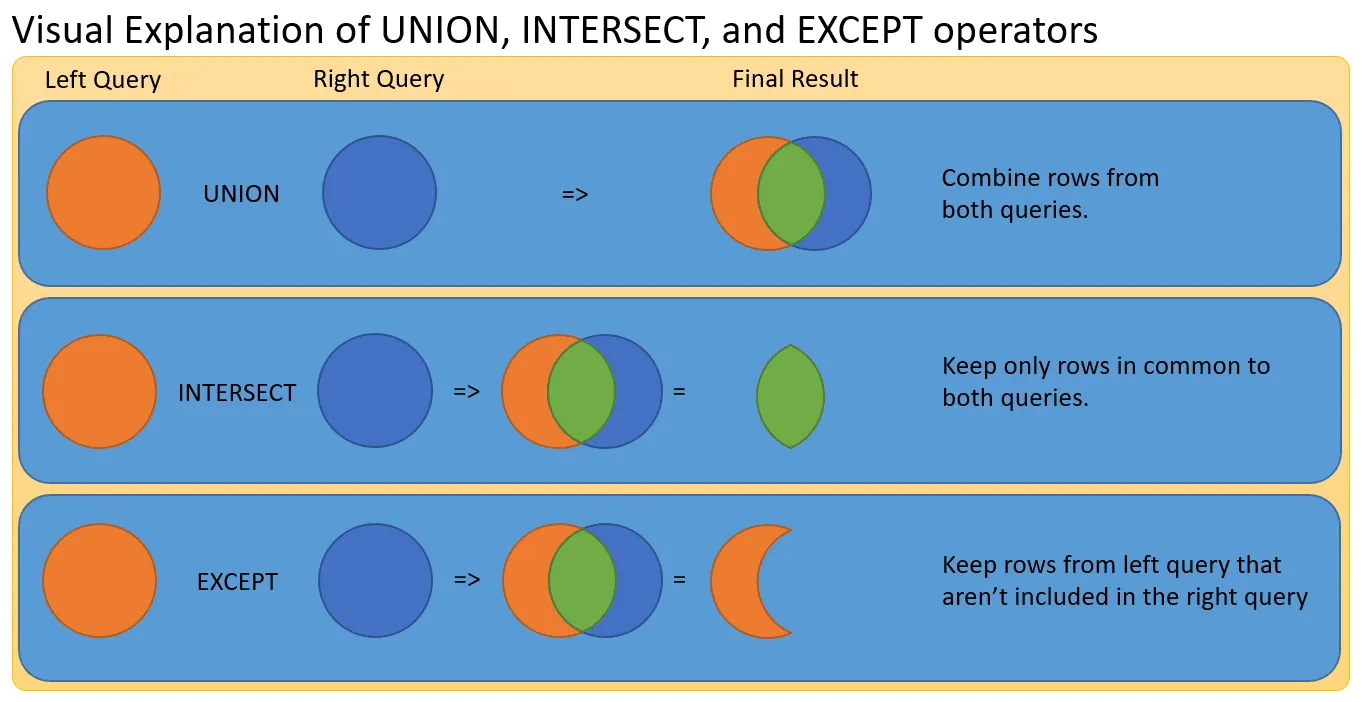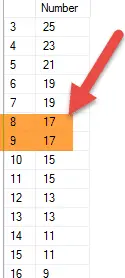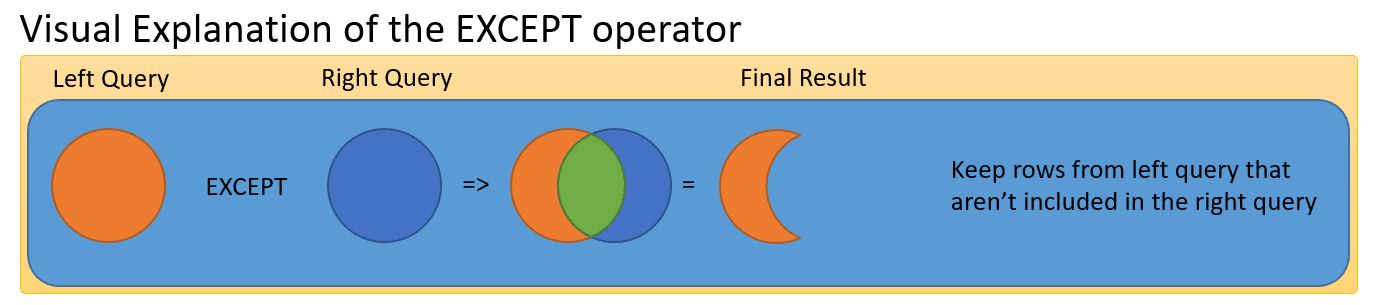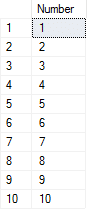# SQL Server and Set Operations

In this puzzle, we’re going to about SQL set operations.  Set operations allow us to compare rows from two or more tables to arrive at a result.   For several classes of problems, is is much easier to use a set operation, than join data.

Solving puzzles is a great way to learn SQL.  Nothing beats practicing what you’ve learned.  Once you have figured out the puzzle, post you answer in the comments so we all can learn from one another.  We also discuss puzzle and more in Essential SQL Learning Group on Facebook.  Be sure to find us there!

## SQL Set Operations Puzzle – Part I

Given two lists of numbers: A and B

1. Select all odd numbers ordered from highest to lowest
2. Select all numbers from A that aren’t in B
3. Select All numbers common to A and B

*** Don’t use joins or subqueries to answer these questions.

Before we get into the answers I think it is important for you to know the difference between a join and set operation.

Though both concepts are used to combine data from multiple tables, join combine columns from separate tables; whereas, set operations combine rows from separate tables.

For instance, union is used to combine rows from two or more table in to a single result.  It is difficult to do this using JOIN.

Today to answer the question, we are going to use UNION, EXCEPT, and INTERSECT respectively.  Below is a visual summary of these operations:If you’re not familiar with set operations,  I would recommend reading this article on UNION, INTERSECT, and EXCEPT.

### Use a Set Operation to Select all odd numbers ordered from highest to lowest

To do this we’ll combine the results of two queries, one from table A the other from B.  In both case, we want only odd numbers.  These are numbers, that when divided by 2, have a remainder of one.

Once we have a combined set, we’ll order the results.

Here are the tools we’ll need to use:

• % – This is the modulus operator. Well use to to get the remainder when we divide number by 2.  Numbers with a remainder of 1 are odd.
• UNION – This set operator allows us to combine rows from one result set with another if each result has the same number of columns and datatypes.
• ORDER BY DESC – We use the ORDER BY clause to sort values.

The query to get odd number from table A is

```SELECT   Number
FROM     @A
WHERE    Number % 2 = 1```

The modulo operator (%) may seem strange, but is it really handy!

We repeat the same query for table B and combine them using UNION ALL and ORDER the results.

```SELECT   Number
FROM     @A
WHERE    Number % 2 = 1
UNION ALL
SELECT   Number
FROM     @B
WHERE    Number % 2 = 1
ORDER BY Number DESC```

Notice that I used UNION ALL.  This returns every row for the union-ed results, even duplicate values.  The above query returns 20 rows, below are some of the results:If I ran the query without ALL, then only 15 rows are returned; try it!

### Use a Set Operator to Select all numbers From A that aren’t in B

To answer this question we’ll use the EXCEPT operator.   It will limit our result to only those rows that are exclusive to one table.For our purposes, think of table A as the orange table, and B as the blue.  When we do A EXCEPT B, we are going return the orange crescent.  This represents rows exclusive to A.

Here is the query to do so:

```SELECT   Number
FROM     @A
EXCEPT
SELECT   Number
FROM     @B```

In this example, we retrieve all rows from A, they are then compared to all rows from B, and only those rows found in A, but Not B are returned in the result:I’m sure some of you were thinking of other solutions, such as those that use a JOIN or Subquery.

Here is the solution as a subquery:

```SELECT   Number
FROM     @A
WHERE    Number NOT IN (SELECT Number
FROM @B)```

I show you this, so you can understand that there are many ways to solve the same problem!

### Use a Set Operator to Select All Numbers Common to A and B

To find all numbers common between two results we can use the INTERSECT operator.  These are the numbers which “overlap” in our diagram.

Though this was the last question in the puzzle, I think it is the easiest to answer!

```SELECT   Number
FROM     @A
INTERSECT
SELECT   Number
FROM     @B```

Can you think of another way of showing numbers common to both tables?  What about an INNER JOIN?

Here is another way to get the same result:

```SELECT   A.Number
FROM     @A A
INNER JOIN @B B
ON A.Number = B.Number```

Also, if you have any ideas for more puzzles, let me know.

#### Kris Wenzel

Kris Wenzel has been working with databases over the past 30 years as a developer, analyst, and DBA. He has a BSE in Computer Engineering from the University of Michigan and a MBA from the University of Notre Dame. Kris has written hundreds of blog articles and many online courses. He loves helping others learn SQL.

• […] a valid expression. For example, you can use CASE in statements such as SELECT, UPDATE, DELETE and SET, and in clauses such as select_list, IN, WHERE, ORDER BY, and […]